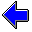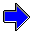Java Security Settings:
This web page employs Java, which requires specific security settings for correct operation.
If the applets on this page do not run correctly, consult the Virtual Chemistry Experiments FAQ
or the Physlet Physics web site for establishing the correct security settings.

# Calorimetry

## Heat of Solution of Ammonium Nitrate

### Concepts

Calorimeters are designed to be well-insulated, so no heat is gained from or lost to the surroundings. If no heating element is used to introduce heat in the system, the total heat (q) for the entire calorimeter system must equal zero. The total heat can be split into heats for each component in the system.

Imagine a reaction in which solid ammonium nitrate (a component in some fertilizers and an explosive) is dissolved in water to produce an aqueous ammonium nitrate solution.

NH4NO3 (s)   →   NH4+ (aq) + NO3- (aq)

The heat flow (qrxn) for this reaction is called the heat of solution for ammonium nitrate. When the reaction is finished, the system contains two substances, the calorimeter itself and the aqueous solution, and there is a heat associated with each component. The heat balance for this experiment is thus

0 = q = qrxn + qcal + qsoln

The basic strategy in calorimetry is to use a temperature change and a heat capacity to determine a heat flow. In this experiment all substances have the same initial and final temperatures.

qcal = Ccal ΔT = Ccal ( Tf - Ti )

qsoln = Csoln
ΔT = msoln ssoln ( Tf - Ti )

One typically determines the heat capacity of the aqueous solution (Csoln) from the mass of the solution (msoln) and the specific heat capacity of the solution (ssoln). The mass of the solution is the sum of the masses of the water and ammonium nitrate originally placed in the calorimeter. The specific heat capacity of the aqueous solution is usually close to that of pure water (4.184 J oC-1 g-1).

The objective of this experiment is to determine the heat of reaction (in this case a heat of solution). The above equations can be combined and rearranged to yield a working equation:

qrxn = - qcal - qsoln = - ( Ccal + msoln ssoln ) ( Tf - Ti )

Just as the heat capacity of a substance is an extensive property, so the heat of solution is an extensive property. It is generally more convenient to report intensive properties, thus the heat capacity of a substance is usually reported as a specific heat capacity, that is, the heat capacity per gram of substance. Similarly one can report a specific heat of solution, which is the heat a solution per gram of solute. More commonly, though, the molar heat of solution is reported. The molar heat of solution (ΔHsoln) is the heat of solution (qrxn) per mole of solute (n).

 ΔHsoln = qrxnn

### Experiment

Objective:

• Determine the molar heat of solution of ammonium nitrate.

Approach:

• Dissolve a known mass of ammonium nitrate in a known mass of water in a calorimeter.
• Observe the temperature of the system before and after the dissolution reaction occurs.
• Calculate the change in temperature for the system.
• Use the temperature change and heat capacities for the calorimeter and aqueous solution to calculate the heat of solution.
• Divide the heat of solution by the moles of ammonium nitrate to obtain the molar heat of solution of NaCl.

### Part 1

In this part of the experiment, the calorimeter is filled with 60.0 g of water. A small sealed glass bulb containing 5.00 g of pure NH4NO3 is placed in the calorimeter. The reaction is initiated by breaking the glass bulb, allowing the NH4NO3 to dissolve in the water.

The heat capacity of the calorimeter (Ccal) is 153. J oC-1. The formula weight of NH4NO3 is 80.04.

### Part 2

Repeat the procedure in Part 1 for determining the heat of solution of ammonium nitrate using different values for the mass of ammonium nitrate and/or the mass of water. You should obtain the same heat of solution as you did in Part 1.

 Mass of NH4NO3 g Mass of Water gSpecific Heat Capacity of Copper                     Heat of NeutralizationCalorimetry Home PageVirtual Chemistry Home Page

HeatOfSolutionOfAmmoniumNitrate.html version 2.11